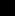# RELATIONS AND FUNCTIONS

## 4.1 RELATIONS

A relation is an association between objects. A book on a table is an example of the relation of one object being on another. It is especially common to speak of relations among people. For example, one person could be the niece of another. In mathematics, there are many relations such as equals and less-than that describe associations between numbers. To formalize this idea, we make the next definition.DEFINITION 4.1.1

A set R is an (n-ary) relation if there exist sets A0, A1, ... , An−1 such thatIn particular, R is a unary relation if n = 1 and a binary relation if n = 2. If RA × A for some set A, then R is a relation on A and we write (A, R).

The relation on can be represented as a subset of the Cartesian product of the set of all books and the set of all tables. We could then write (dictionary, desk) to mean that the

dictionary is on the desk. Similarly, the set {(2, 4), (7, 3), (0, 0)} is a relation because it is a subset of Z × Z. The ordered pair (2, 4) means that 2 is related to 4. Likewise, R × Q is a relation where every real number is related to every rational number, and according to Definition 4.1.1, the empty set is also a relation because ∅ = ∅ × ∅.

EXAMPLE 4.1.2

For any set A, define

Call this set the identity ...

Get A First Course in Mathematical Logic and Set Theory now with the O’Reilly learning platform.

O’Reilly members experience books, live events, courses curated by job role, and more from O’Reilly and nearly 200 top publishers.